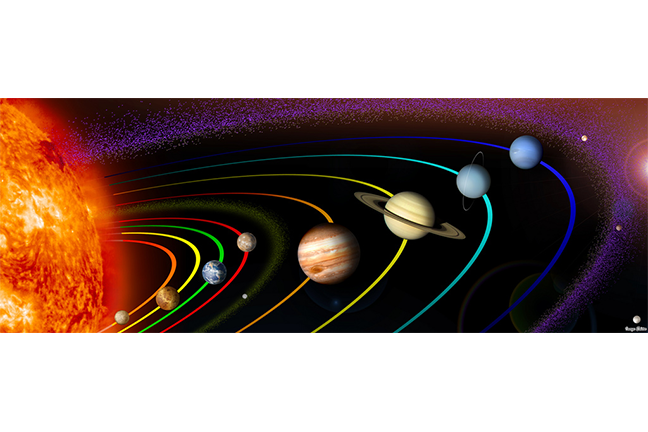4.8

## UNSW SydneyEight ellipses

# Kepler's Third Law: the law of harmonies

In 1609 Kepler formulated his first two laws to summarize the remarkable astronomical observations his mentor Tycho Brahe had made over many years. Ten years later he published his third law, sometimes called the law of harmonies. It is a beautiful example of a power law between the period of an orbiting body and its average distance to the body it orbits.

In this step we

• look at data about the motion of the planets

• explain Kepler’s third law

• investigate whether the law of harmonies also holds for satellites of the earth.

## Periods and average distances of planets

Most planets have elliptical orbits — this is Kepler’s First Law, with the added provision that in the case of a small planet revolving around a big sun, the sun will be at one of the foci of the elliptic orbit of the planet. In fact most orbits are quite close to being circles.

Kepler’s Second Law asserts that areas swept out by a planet in equal times are equal. Kepler’s Third Law is the most complicated, and it relates the period $\normalsize{T}$ of a planet, which is the time spent for one revolution around the sun, to the average distance $\normalsize{R}$ to the sun.

The units for both quantities are taken from the earth’s orbit: period is measured in years, which is the time taken for the earth to complete one of its orbits, and distance is taken in astronomical units (au), which is the average distance from the earth to the sun. This turns out to be about $\normalsize{150}$ million kilometres.

So for the earth $\normalsize{T=1}$ and $\normalsize{R=1}$ by definition.

The question that Kepler answered was: what is the general relation between $\normalsize{R}$ and $\normalsize{T}$ for the other planets?

## Kepler’s third law

Kepler’s discovery was that the period $\normalsize{T}$ and the average distance $\normalsize{R}$ of a planet from the sun obeyed the relation

Or if we want to find $\normalsize{T}$ from $\normalsize{R}$, the expression is

This is a power law, but now with an exponent bigger than $\normalsize{1}$. Here is the graph of $\normalsize{y=x^\frac{3}{2}}$:## The data

Here is a table of periods and average distances for the planets, as well as the ratio $\normalsize{T^2/R^3}$.

Planet Period (yr) Average Distance (au) $\normalsize{T^2/R^3}$
Mercury 0.241 0.39 0.98
Venus .615 0.72 1.01
Earth 1.00 1.00 1.00
Mars 1.88 1.52 1.01
Jupiter 11.8 5.20 0.99
Saturn 29.5 9.54 1.00
Uranus 84.0 19.18 1.00
Neptune 165 30.06 1.00
Pluto 248 39.44 1.00

Our source for this data is the Kepler’s three laws page over at the physicsclassroom.com.

## The more general form of the Third Law

More generally, if several satellites orbit the same more massive object, then the period $\normalsize{T}$ of an orbiting body will relate to the mean distance $\normalsize{R}$ from the center of the massive object according to the power law

where $\normalsize{A}$ is a constant that depends only on the massive object. In particular this law holds for other objects orbiting the sun, and also holds for satellites orbiting the earth. However in this latter case the constant $\normalsize{A}$ will not be $\normalsize{1}$.

## The case of comets

Comets which are recurrent also obey Kepler’s law. The most famous one is Halley’s comet, with an orbital period of $\normalsize{75.3}$ years and an average distance of $\normalsize{17.55}$ au.

You can compute that

which is pretty close to one.Lspn comet halley By NASA/W. Liller, Public domain, via Wikimedia Commons

Q1 (E): Jupiter has four moons. They also obey Kepler’s laws in their orbits around Jupiter. The distance of the moon called Io from Jupiter’s centre is $\normalsize{4.2}$ units, and its period is $\normalsize{1.8}$ Earth-days. Another moon is called Ganymede; it is $\normalsize{10.7}$ units from Jupiter’s center. What is the period of Ganymede (pictured)?Ganymede moon By NASA/JPL/DLR (Ganymede’s Trailing Hemisphere), Public domain, via Wikimedia Commons

## The moon and other satellites of earth

What about our moon? That is also an orbiting body, but this time orbiting around the earth. It is known to be about 384,400 km from the earth, as compared to the distance from the earth to the sun of one a.u., or about 150 million km. The period of the moon is about 27.32 days. So let’s calculate what the ratio $\normalsize T^2/R^3$ is in units of $\normalsize yr^2/au^3$ for the moon revolving around the earth. Some conversions are needed – but we are experts at that!

In units of earth years, the orbital period of the moon is

In astronomical units the mean distance of the moon from the earth (centre to centre) is

Putting these together, we get that

The geostationary satellites are exactly at the correct height to ensure that their orbit times are exactly one day. This guarantees that they are constantly above a fixed equatorial location on the earth, as it also rotates around its axis once a day, so that their periods in years are

Measured from the center of the earth the height of such a satellite is $\normalsize 42,164$ kilometres. So in a.u. units this is

Putting these together we get

These two values for the moon and a geostationary satellite are reasonably close together, giving further evidence that Kepler’s power law relation also holds for general orbiting bodies.

## Discussion

What about for the International Space Station? Can you gather some data, and make some calculations, and see if that also roughly fits with Kepler’s law of harmonies in its orbit around the earth?

A1. Kepler’s third law says that $\normalsize T^2/R^3$ should be roughly the same for all bodies orbiting Jupiter. So if we call Ganymede’s orbital period $\normalsize T_G$ then by comparing with Io’s orbit, we must have
Solving, we get $\normalsize T_G\approx 7.3$ Earth-days. The actual value is $\normalsize 7.155$ Earth-days.